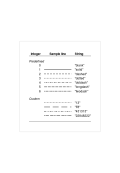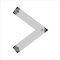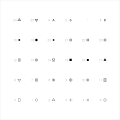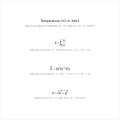Figure 10.1 Predefined and custom line typesR code
 Figure 10.2 Line join and line ending stylesR code
 Figure 10.3 Data symbols available in RR code
 Figure 10.4 Mathematical formulae in plotsR code